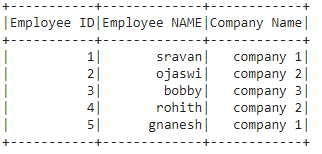# How to check the schema of PySpark DataFrame?

• Last Updated : 17 Jun, 2021

In this article, we are going to check the schema of pyspark dataframe. We are going to use the below Dataframe for demonstration.Method 1: Using df.schema

Schema is used to return the columns along with the type.

Syntax: dataframe.schema

Where, dataframe is the input dataframe

Code:

## Python3

 `# importing module``import` `pyspark`` ` `# importing sparksession from pyspark.sql module``from` `pyspark.sql ``import` `SparkSession`` ` `# creating sparksession and giving an app name``spark ``=` `SparkSession.builder.appName(``'sparkdf'``).getOrCreate()`` ` `# list  of employee data with 5 row values``data ``=` `[[``"1"``, ``"sravan"``, ``"company 1"``],``        ``[``"2"``, ``"ojaswi"``, ``"company 2"``],``        ``[``"3"``, ``"bobby"``, ``"company 3"``],``        ``[``"4"``, ``"rohith"``, ``"company 2"``],``        ``[``"5"``, ``"gnanesh"``, ``"company 1"``]]`` ` `# specify column names``columns ``=` `[``'Employee ID'``, ``'Employee NAME'``, ``'Company Name'``]`` ` `# creating a dataframe from the lists of data``dataframe ``=` `spark.createDataFrame(data, columns)`` ` `# display dataframe columns``dataframe.schema`

Output:

```StructType(List(StructField(Employee ID,StringType,true),
StructField(Employee NAME,StringType,true),
StructField(Company Name,StringType,true)))```

Method 2: Using schema.fields

It is used to return the names of the columns

Syntax: dataframe.schema.fields

where dataframe is the dataframe name

Code:

## Python3

 `# importing module``import` `pyspark`` ` `# importing sparksession from pyspark.sql module``from` `pyspark.sql ``import` `SparkSession`` ` `# creating sparksession and giving an app name``spark ``=` `SparkSession.builder.appName(``'sparkdf'``).getOrCreate()`` ` `# list  of employee data with 5 row values``data ``=` `[[``"1"``, ``"sravan"``, ``"company 1"``],``        ``[``"2"``, ``"ojaswi"``, ``"company 2"``],``        ``[``"3"``, ``"bobby"``, ``"company 3"``],``        ``[``"4"``, ``"rohith"``, ``"company 2"``],``        ``[``"5"``, ``"gnanesh"``, ``"company 1"``]]`` ` `# specify column names``columns ``=` `[``'Employee ID'``, ``'Employee NAME'``, ``'Company Name'``]`` ` `# creating a dataframe from the lists of data``dataframe ``=` `spark.createDataFrame(data, columns)`` ` `# display dataframe columns``dataframe.schema.fields`

Output:

```[StructField(Employee ID,StringType,true),
StructField(Employee NAME,StringType,true),
StructField(Company Name,StringType,true)]```

Method 3: Using printSchema()

It is used to return the schema with column names

Syntax: dataframe.printSchema()

where dataframe is the input pyspark dataframe

## Python3

 `# importing module``import` `pyspark`` ` `# importing sparksession from pyspark.sql module``from` `pyspark.sql ``import` `SparkSession`` ` `# creating sparksession and giving an app name``spark ``=` `SparkSession.builder.appName(``'sparkdf'``).getOrCreate()`` ` `# list  of employee data with 5 row values``data ``=` `[[``"1"``, ``"sravan"``, ``"company 1"``],``        ``[``"2"``, ``"ojaswi"``, ``"company 2"``],``        ``[``"3"``, ``"bobby"``, ``"company 3"``],``        ``[``"4"``, ``"rohith"``, ``"company 2"``],``        ``[``"5"``, ``"gnanesh"``, ``"company 1"``]]`` ` `# specify column names``columns ``=` `[``'Employee ID'``, ``'Employee NAME'``, ``'Company Name'``]`` ` `# creating a dataframe from the lists of data``dataframe ``=` `spark.createDataFrame(data, columns)`` ` `# display dataframe columns``dataframe.printSchema()`

Output:

```root
|-- Employee ID: string (nullable = true)
|-- Employee NAME: string (nullable = true)
|-- Company Name: string (nullable = true)```

My Personal Notes arrow_drop_up